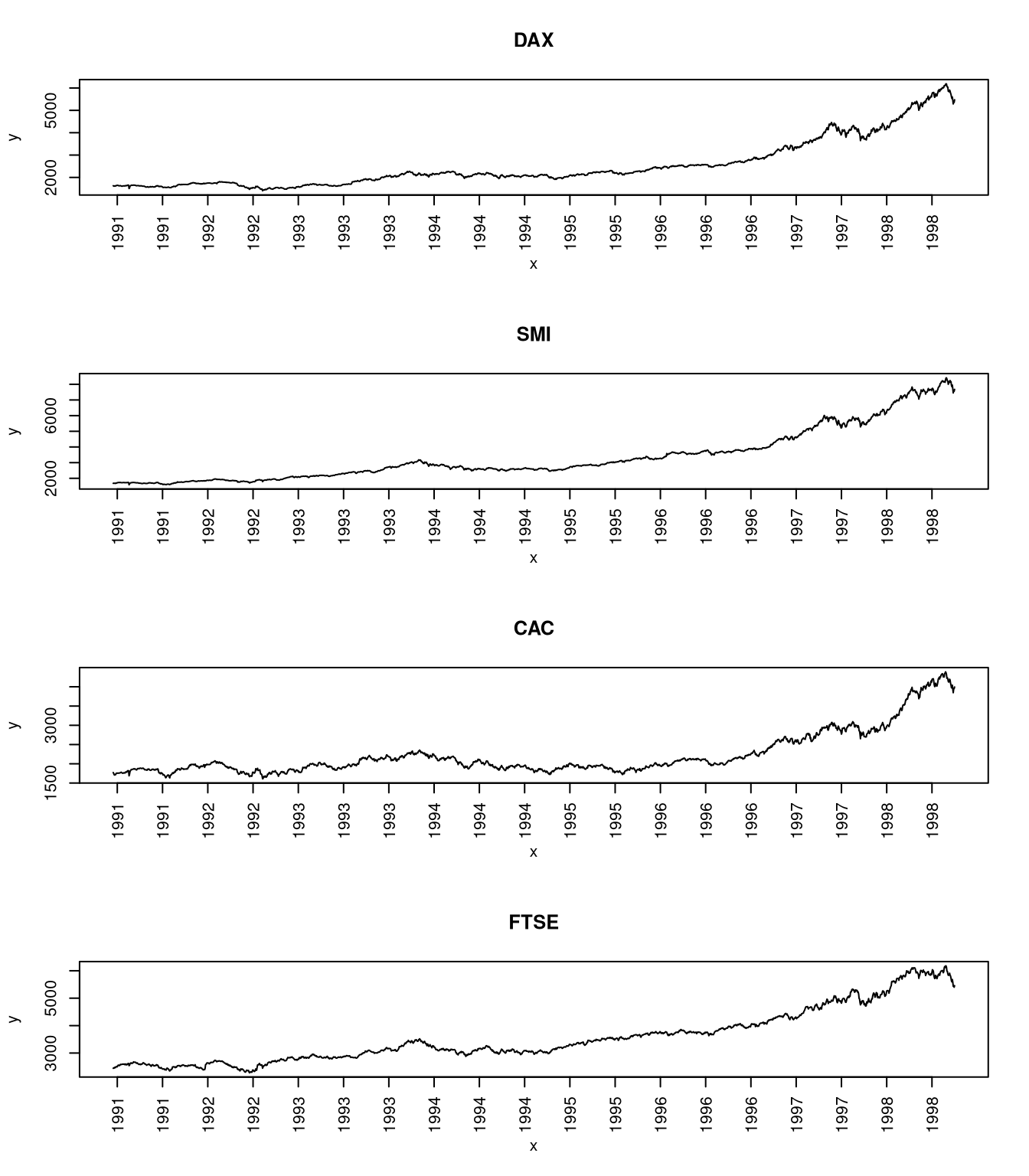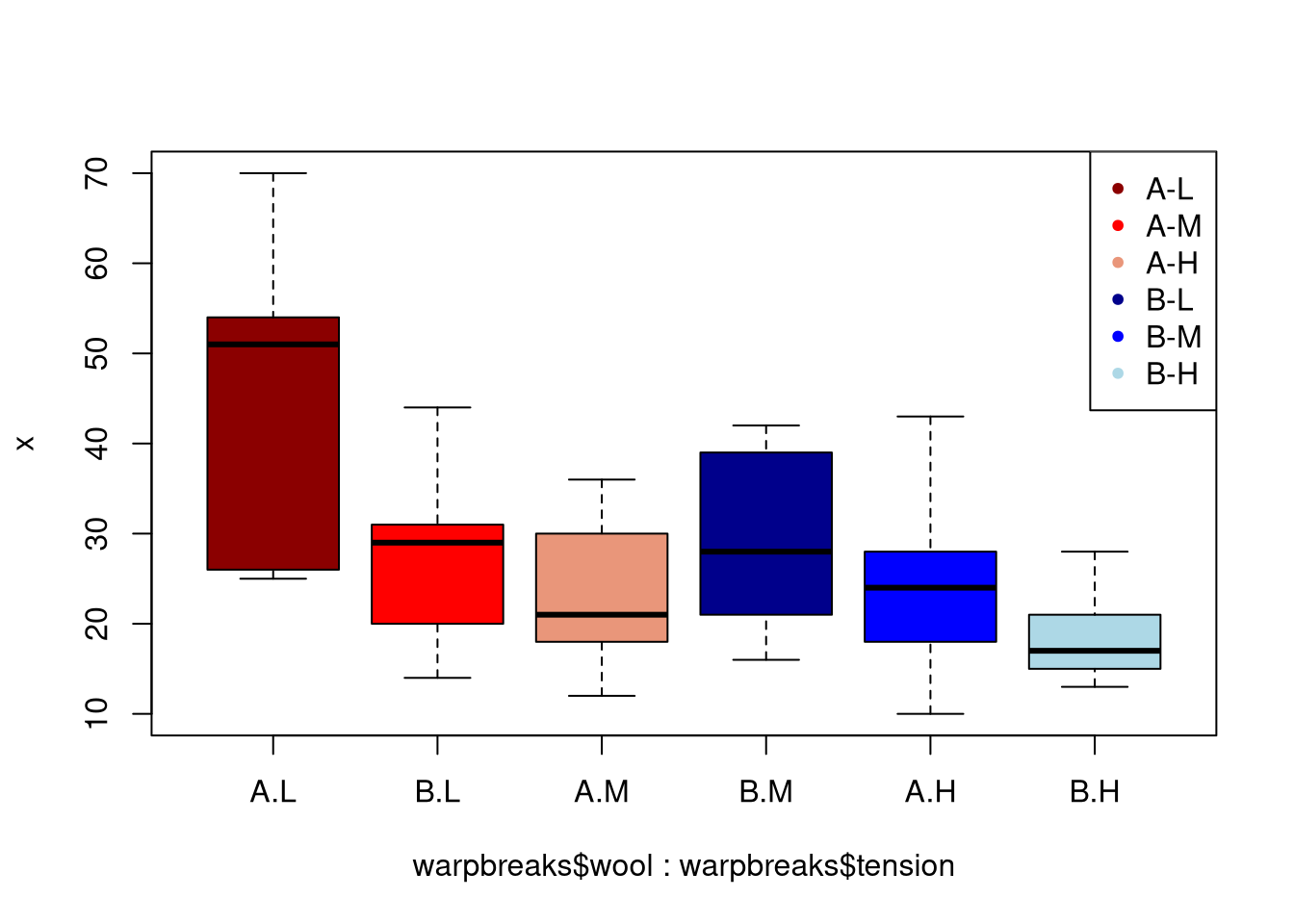# Articles by R on datascienceblog.net: R for Data Science

### Linear, Quadratic, and Regularized Discriminant Analysis

November 29, 2018 |

Discriminant analysis encompasses methods that can be used for both classification and dimensionality reduction. Linear discriminant analysis (LDA) is particularly popular because it is both a classifier and a dimensionality reduction technique. Quadratic discriminant analysis (QDA) is a variant of LDA that allows for non-linear separation of data. Finally, regularized ...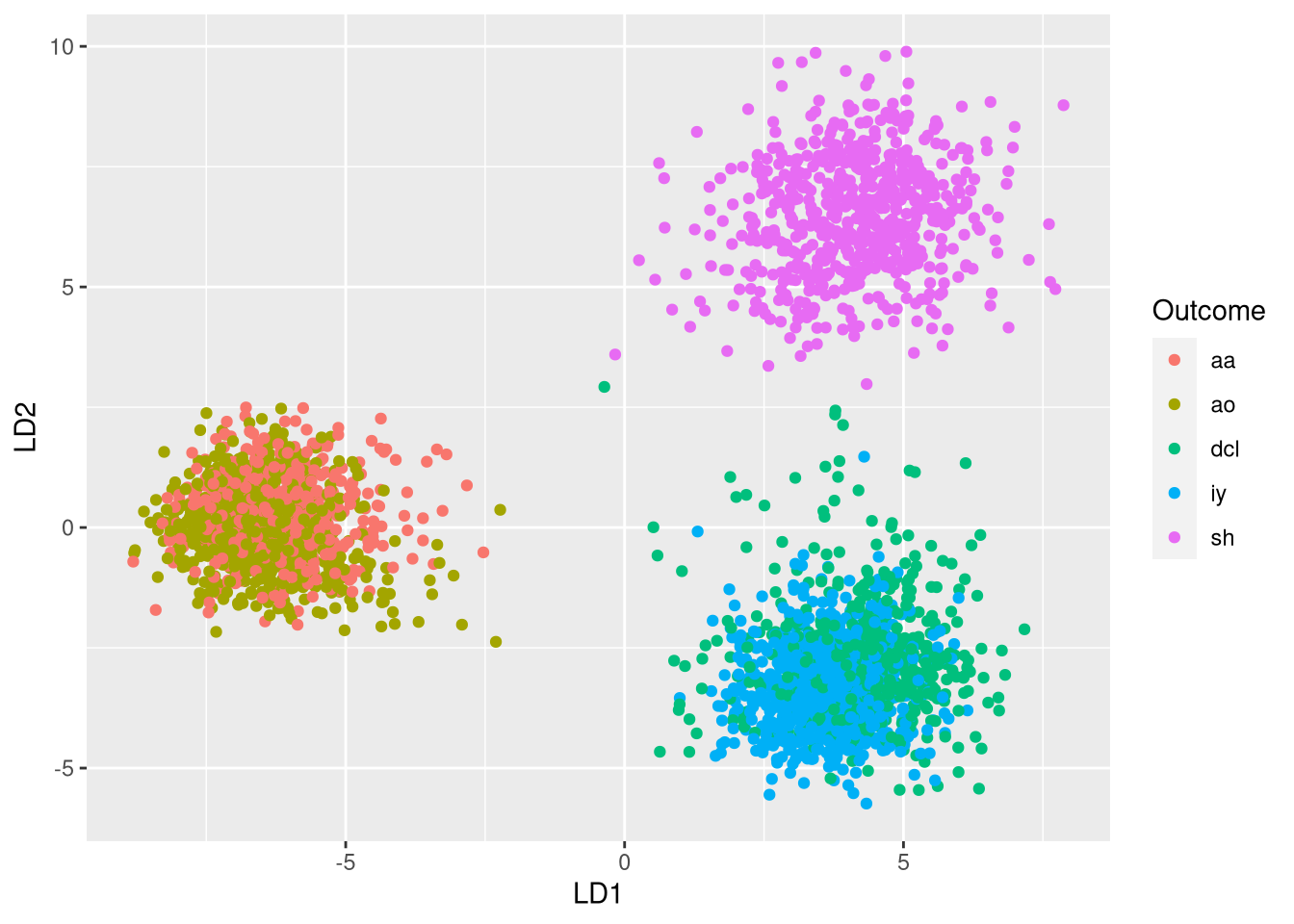### An Introduction to Probabilistic Programming with Stan in R

November 27, 2018 |

Probabilistic programming enables us to implement statistical models without having to worry about the technical details. It is particularly useful for Bayesian models that are based on MCMC sampling. In this article, I investigate how Stan can be used through its implementation in R, RStan. This post is largely based ...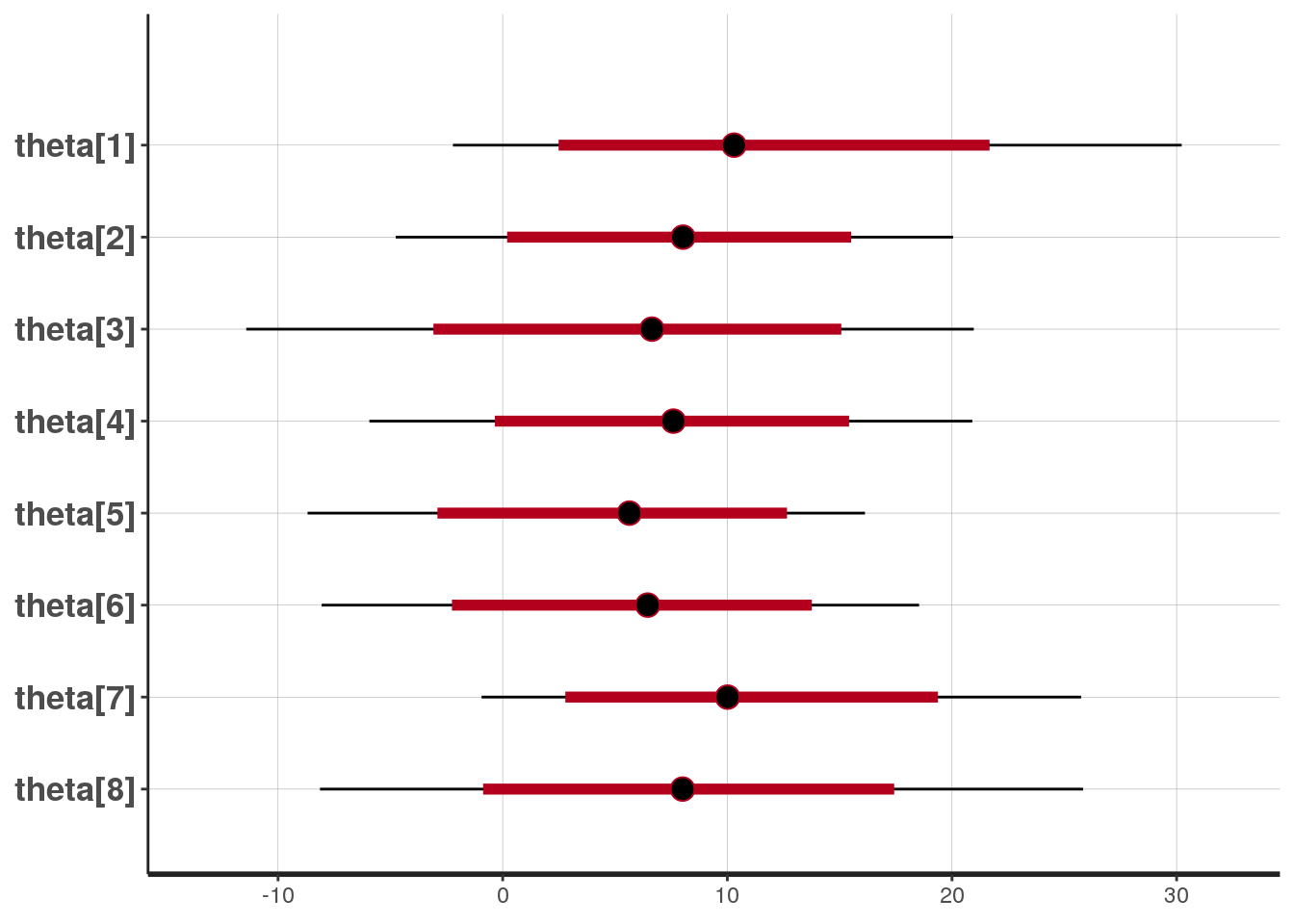### Performance Measures for Feature Selection

November 24, 2018 |

In a recent post, I have discussed performance measures for model selection. This time, I write about a related topic: performance measures that are suitable for selecting models when performing feature selection. Since feature selection is concerned with reducing the number of dependent variables, suitable performance measures evaluate the trade-off ... [Read more...]

### The Case Against Precision as a Model Selection Criterion

November 20, 2018 |

Recently, I have introduced sensitivity and specificity as performance measures for model selection. Besides these measures, there is also the notion of recall and precision. Precision and recall originate from information retrieval but are also used in machine learning settings. However, the use of precision and recall can be problematic ...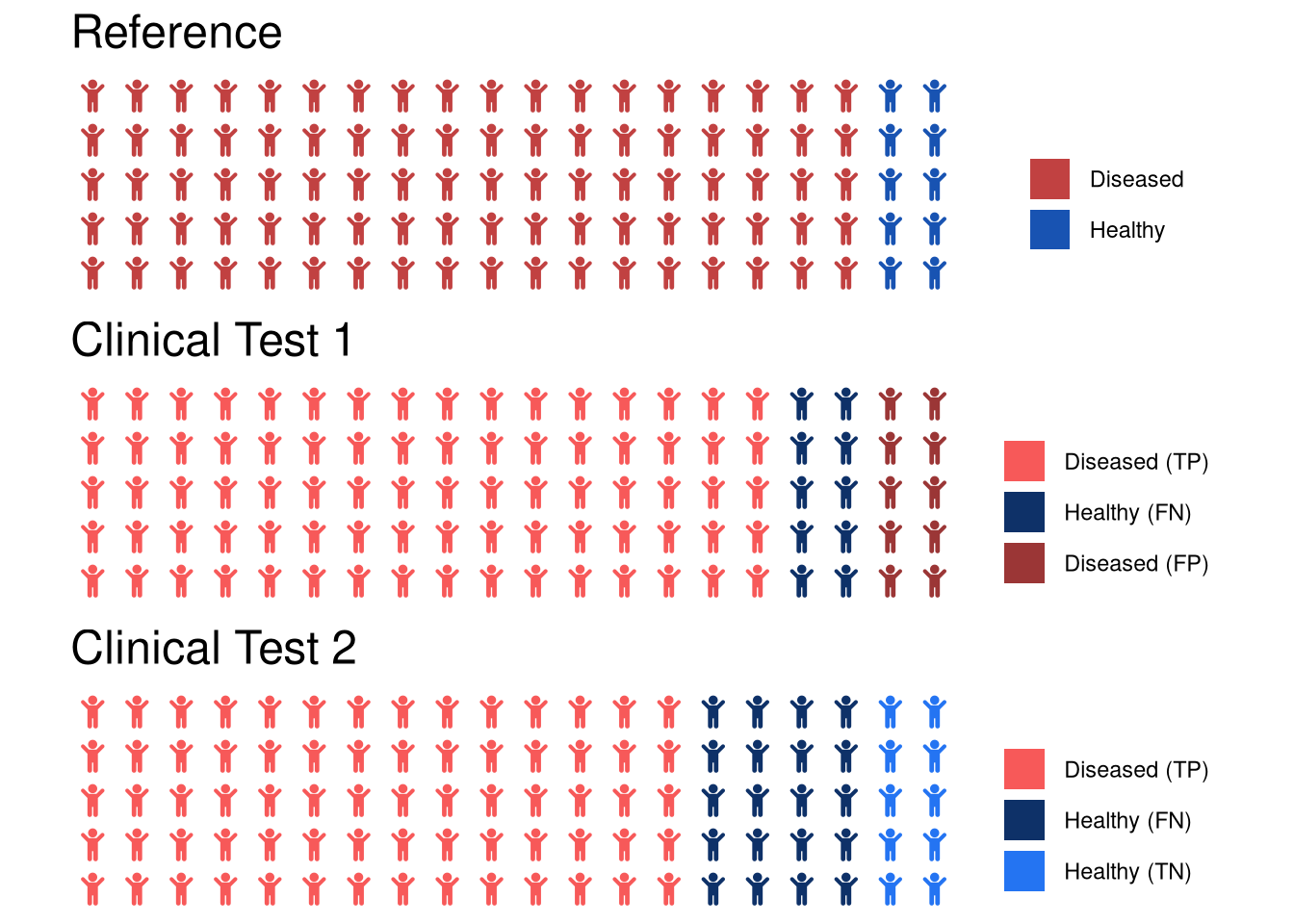### Dimensionality Reduction for Visualization and Prediction

November 13, 2018 |

Dimensionality reduction has two primary use cases: data exploration and machine learning. It is useful for data exploration because dimensionality reduction to few dimensions (e.g. 2 or 3 dimensions) allows for visualizing the samples. Such a visualization can then be used to obtain insights from the data (e.g. detect clusters ...November 12, 2018 |

Radar plots visualize several variables using a radial layout. This plot is most suitable for visualizing and comparing the properties associated with individual objects. In the following, we will use a radar plot for comparing the characteristics of whiskeys from different distilleries. A data set on whiskey Some of you ...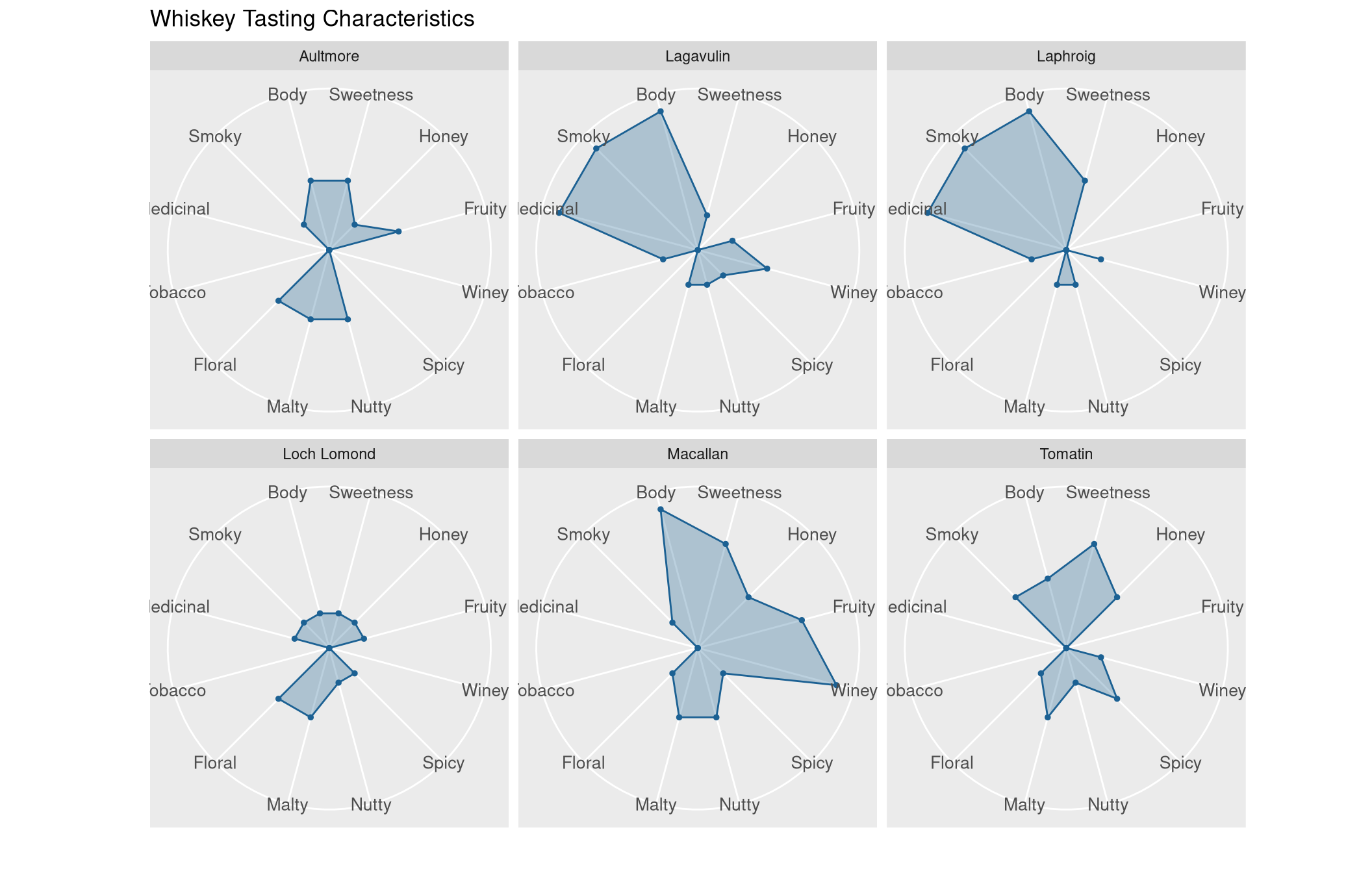### Interpreting Generalized Linear Models

November 9, 2018 |

Interpreting generalized linear models (GLM) obtained through glm is similar to interpreting conventional linear models. Here, we will discuss the differences that need to be considered. Basics of GLMs GLMs enable the use of linear models in cases where the response variable has an error distribution that is non-normal. Each ...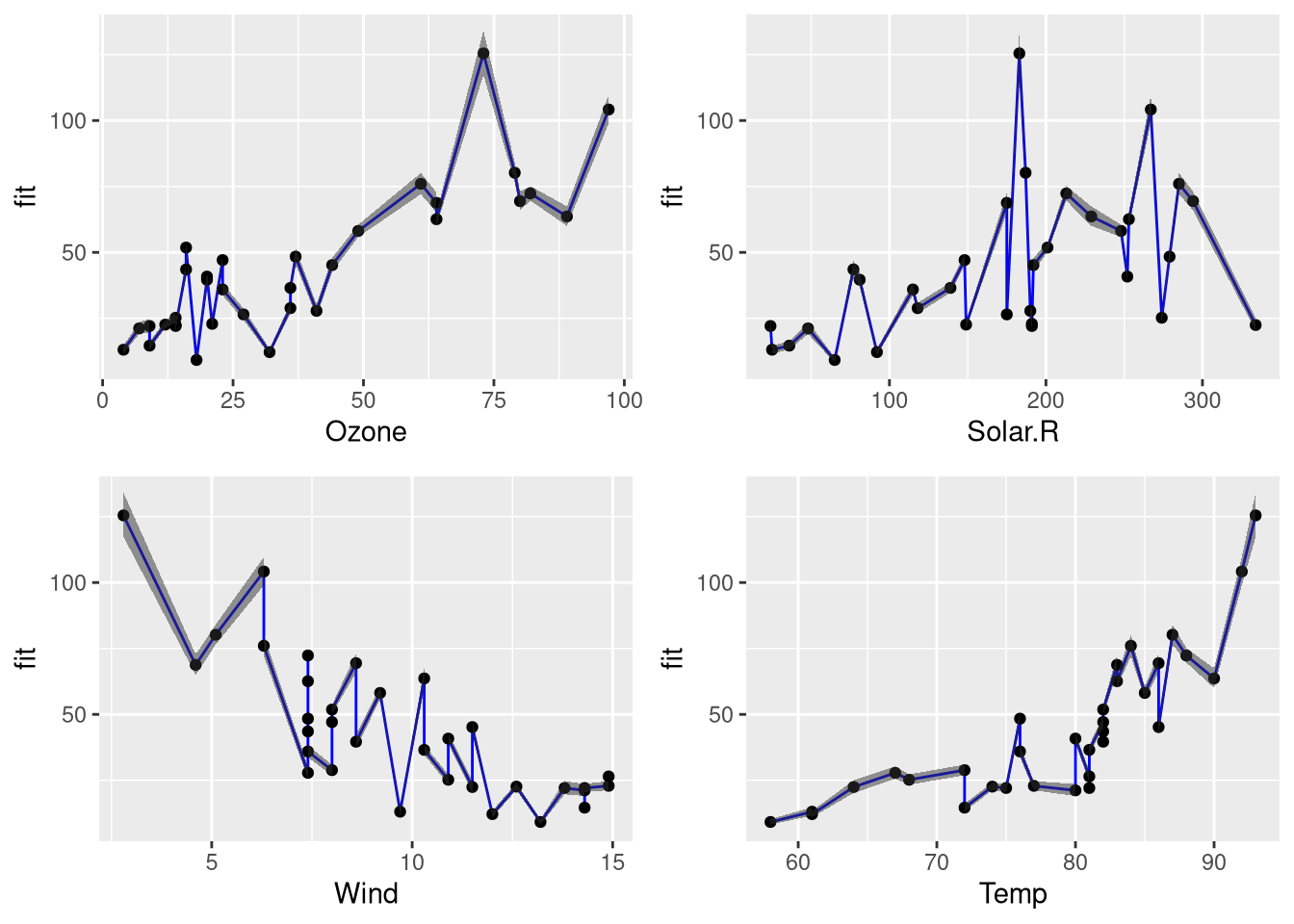### Finding a Suitable Linear Model for Ozone Prediction

November 7, 2018 |

In a previous post, I have introduced the airquality data set in order to demonstrate how linear models are interpreted. In this post, I will start with a basic linear model and, from there, try to find a linear model with a better fit. Data preprocessing Since the airquality data ...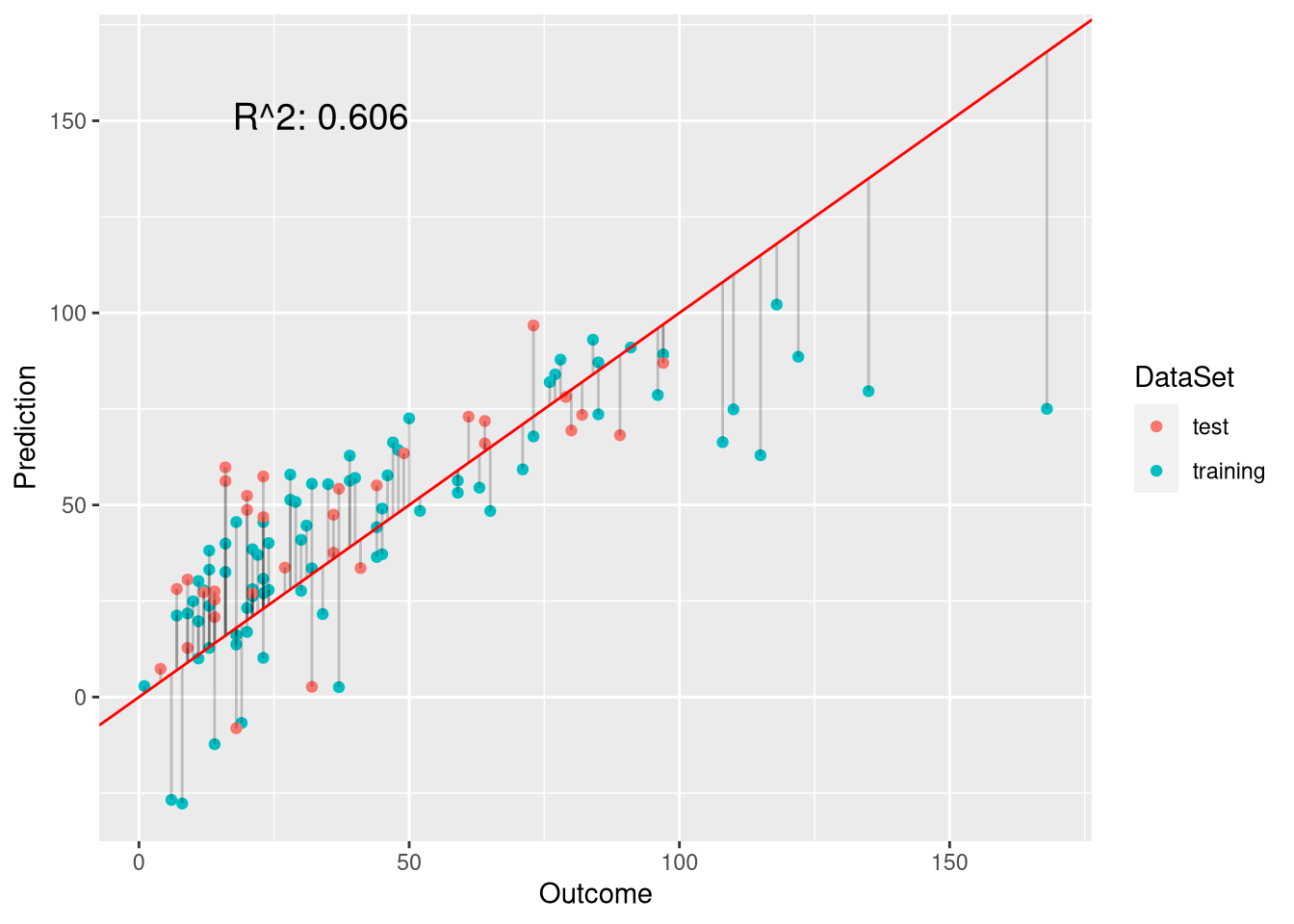### Interpreting Linear Prediction Models

November 6, 2018 |

Although linear models are one of the simplest machine learning techniques, they are still a powerful tool for predictions. This is particularly due to the fact that linear models are especially easy to interpret. Here, I discuss the most important aspects when interpreting linear models by example of ordinary least-squares ...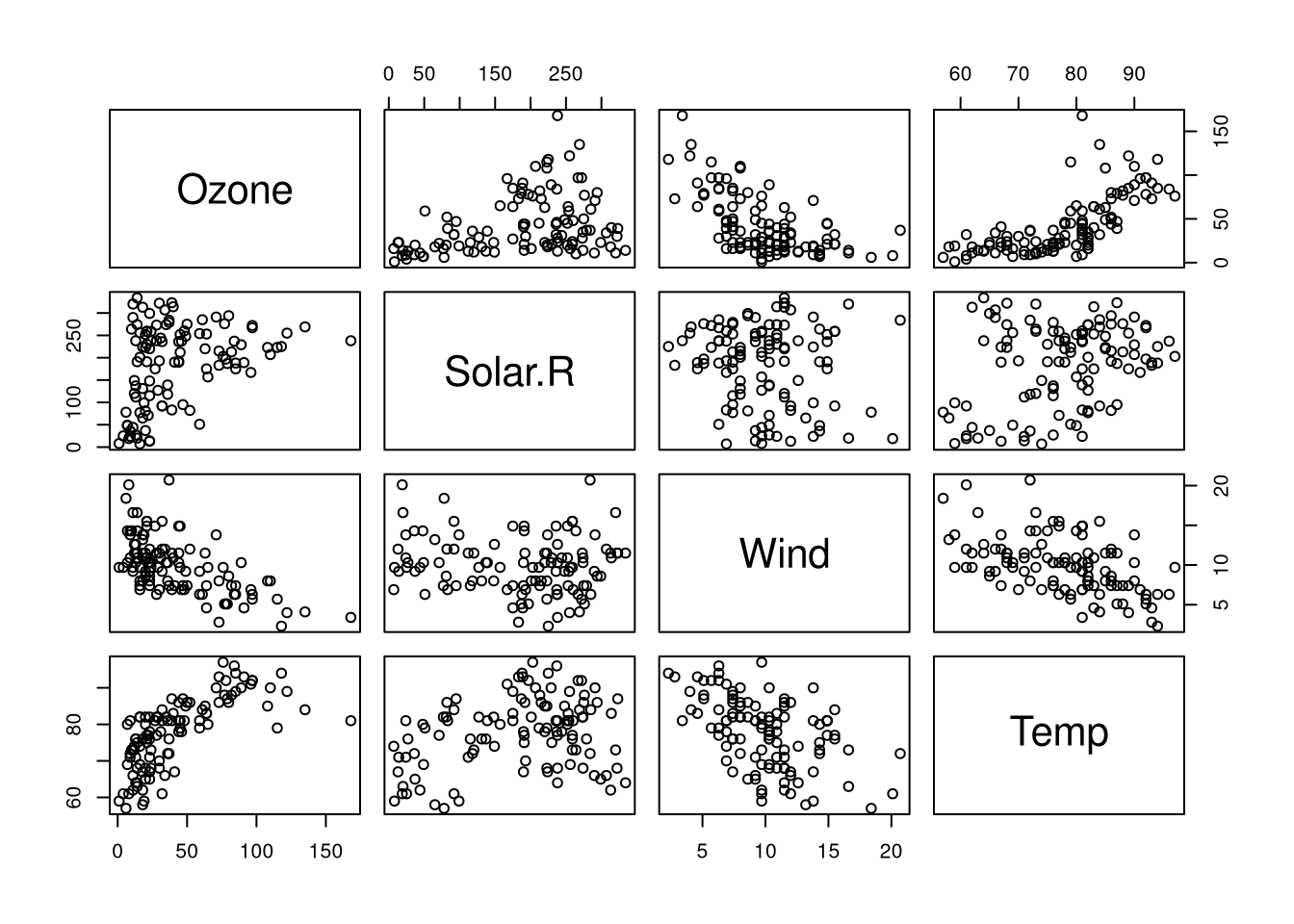### Box Plot Alternatives: Beeswarm and Violin Plots

November 3, 2018 |

Box plots are great as they do not only indicate the median value but also show the variation of the measurements in terms of the 1st and 3rd quartiles. There are, however, also plots that provide a bit of additional information. Here, we take a closer look at potential alternatives ...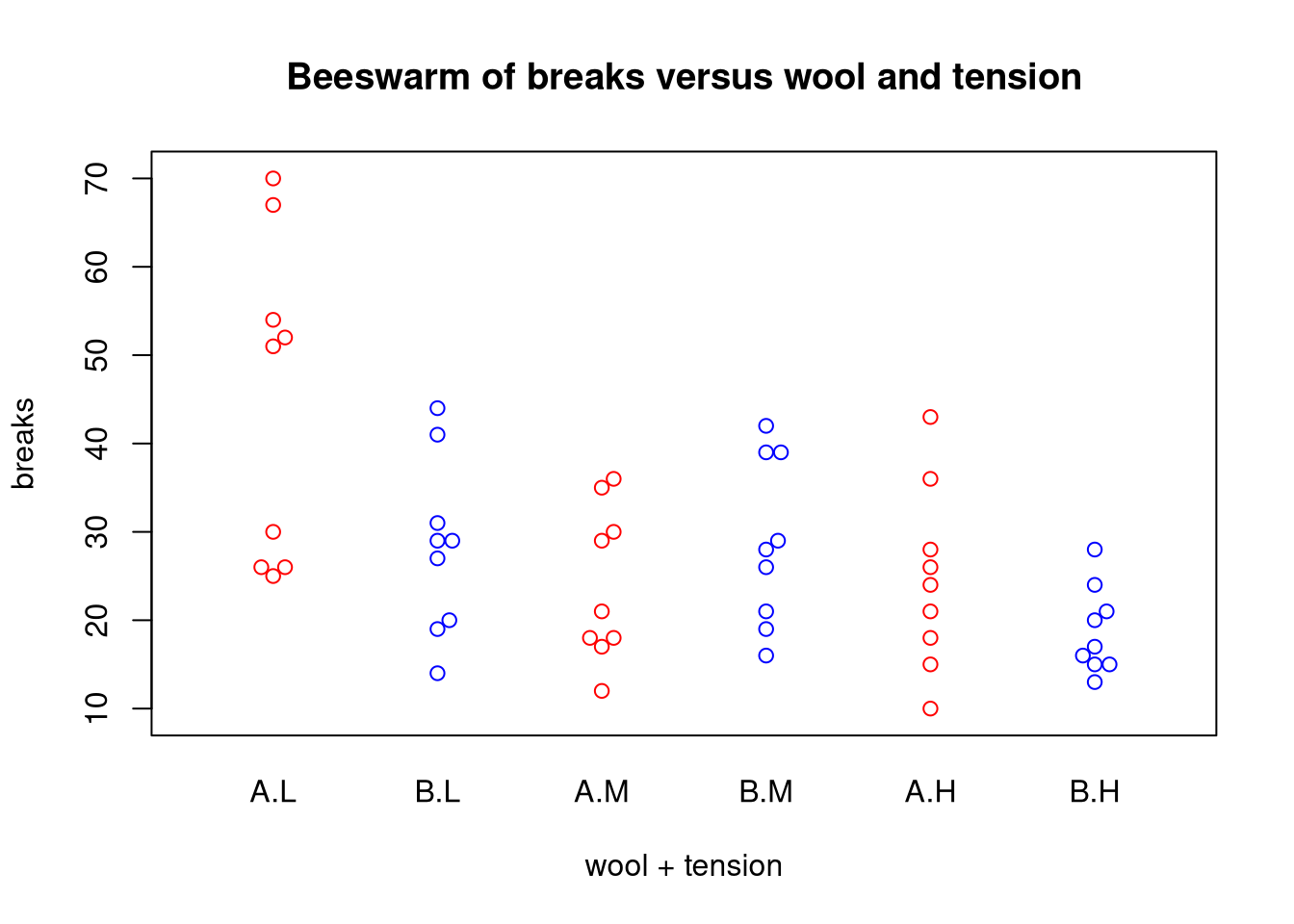### Visualizing Time-Series Data with Line Plots

November 1, 2018 |

The line plot is the go-to plot for visualizing time-series data (i.e. measurements for several points in time) as it allows for showing trends along time. Here, we’ll use stock market data to show how line plots can be created using native R, the MTS package, and ggplot. ...